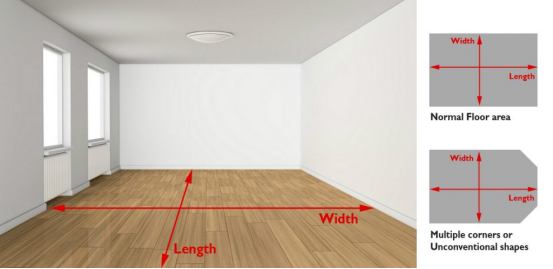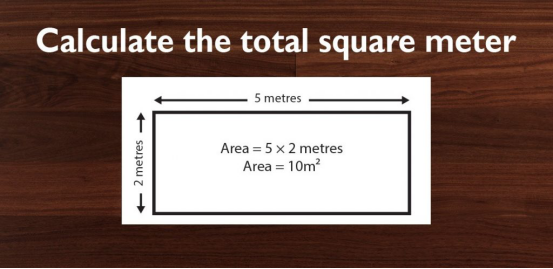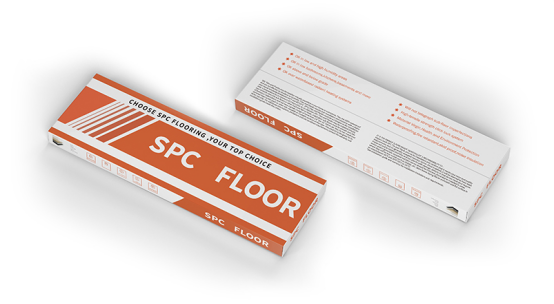# How to calculate the floor area？

Step 1: Understanding your floor space

You can take your own measurement for the floor area.

For multiple corner floor space, measure their farthest point for the length and width.Step 2: Calculate your floor area

Example: 3.85m (Width) X 12m (Length) = 46.2 square meter

Add 10% Wastage for the total floor area.

10% Wastage is needed for cutting wastage of the materials.

Example: 46.2 (floor area) X 1.10 (10% Wastage) = 50.82 square meterStep 3: How many boxes do I need to purchase?

You need to divide the floor area by each box. Example each box cover 1.77 square meter.

50.82 divide by 1.77 = 28.72

So total you need to purchase 29 Boxes。

If each box cost \$55.00 then 29 boxes X \$55.00 = \$1595.00 (Total cost for 29 boxes, supply materials only)Get the latest price? We'll respond as soon as possible(within 12 hours)